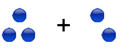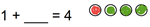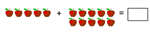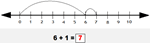Adding with pictures (sums to 10)Addition sentencesAdding with pictures (sums to 30)Number lines:
Adding with number linesAdding 3 numbers with number lines

Add two numbers - sum 10 or less   2 + 6 =
Add two 1-digit numbers - sum 20 or less   9 + 8 =

Number bonds - sums with 8   4 + __ = 8
Number bonds - sums with 9   4 + __ = 9
Number bonds - sums with 10   4 + __ = 10
Adding using combinations of 10   8+5 = 8+2+3=__

Adding doubles   4 + 4 =
Adding doubles plus 1   4 + 5 =
Adding doubles plus 2   4 + 6 =
Adding doubles minus 1   4 + 3 =
Adding doubles and near doubles   6 + 8 =

Missing addends - sum 10 or less   2 + __ = 7
Missing addends - sum 20 or less   2 + __ = 17

Add 3 numbers   3 + 6 + 5 =

Add whole tens   20 + 30 =

___ + 90 = 170

Complete the next ten   86 + ___ = 90
Add 2-digit and 1-digit numbers within the same ten   35 + 4 =

## Adding in columns (no carrying)

Add 2-digit and 1-digit numbers in columns - no carrying

35

+ 4

Add two 2-digit numbers in columns - no carrying

34

+ 15

Add a 2-digit and a 1-digit number in columns - missing addend

26

+__

29

20

+

75

Addition with sums 50 or less

Add / subtract word problems with mostly single digit numbers
Add / subtract word problems with numbers under 50